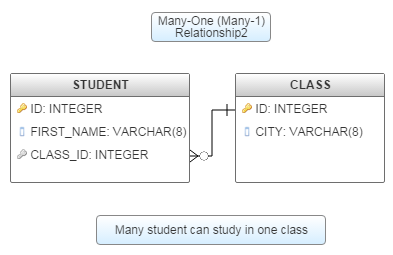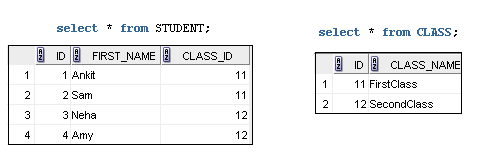### Many - 1 relationship in MongoDB - Many to One

You are here : Home / MongoDB Tutorial

Contents of page >
• 2.2.1) Many-one (Many-1) Relationship - Table structure in RDBMS >
• 2.2.2) Sql script to create above tables in RDBMS (in oracle) >
• 2.2.3) Let’s see tables after inserting data in RDBMS >
• 3.1) create and insert in STUDENT collection (Also insert related data i.e. data (documents) of CLASS in STUDENT collection) >

1) (Many-to-1) Many-to-One Relationships with Embedded document >

Embed the CLASS’s documents in the student documents.
It helps in fetching all student and CLASS data in one query.
But, this is not a good approach, here you can see too much redundant data (_id = 1 and 2 are having same class document, same is the case with _id = 3 and 4), you can go for it till the data is less, as the data grows you will need to follow some normalized approach as shown below (where documents in student contain a reference to the CLASS document).

1.1) Create collection in MongoDB >

STEP 1.1.1) create and insert in STUDENT collection >
 db.STUDENT.insert({  "_id": 1,  "FIRST_NAME": "Ankit",  "CLASS": {"CLASS_NAME": "FirstClass"}}) db.STUDENT.insert({  "_id": 2,  "FIRST_NAME": "Sam",  "CLASS": {"CLASS_NAME": "FirstClass"}}) db.STUDENT.insert({  "_id": 3,  "FIRST_NAME": "Neha",  "CLASS": {"CLASS_NAME": "SecondClass"}}) db.STUDENT.insert({  "_id": 4,  "FIRST_NAME": "Amy",  "CLASS": {"CLASS_NAME": "SecondClass"}})

1.2 > Now, let’s read/query/find in above MongoDB collection >

Query 1.2.1 > Query to show all students
 db.STUDENT.find().pretty()

Output>
 {        "_id" : 1,        "FIRST_NAME" : "Ankit",        "CLASS" : {                "CLASS_NAME" : "FirstClass"        } } {        "_id" : 2,        "FIRST_NAME" : "Sam",        "CLASS" : {                "CLASS_NAME" : "FirstClass"        } } {        "_id" : 3,        "FIRST_NAME" : "Neha",        "CLASS" : {                "CLASS_NAME" : "SecondClass"        } } {        "_id" : 4,        "FIRST_NAME" : "Amy",        "CLASS" : {                "CLASS_NAME" : "SecondClass"        } }

Query 1.2.2 > Query to Find student with FIRST_NAME=”Ankit”
 db.STUDENT.find({"FIRST_NAME":"Ankit"}).pretty()

Output>
 {        "_id" : 1,        "FIRST_NAME" : "Ankit",        "CLASS" : {                "CLASS_NAME" : "FirstClass"        } }

2) (Many-to-1) Many-to-One Relationships with Document Reference (BEST APPROACH)  >

This most normalized approach to model many to one relationship.

This is a good and best approach, here you can see no redundant data (_id = 1 and 2 are having reference of same class document, same is the case with _id = 3 and 4), you can go for when data is huge, it's the most normalized approach to model data in many-to-one relationship.
Create CLASS collection and student collection.
Where documents in student contain a reference to the CLASS document.
2.1) Create collections in MongoDB >

STEP 2.1.1) create and insert in CLASS collection >
 db.CLASS.insert({  "_id": 11,  "CLASS_NAME": "FirstClass"}) db.CLASS.insert({  "_id": 12,  "CLASS_NAME": "SecondClass"})

STEP 2.1.2) create and insert in STUDENT collection >
 db.STUDENT.insert({  "_id": 1,  "FIRST_NAME": "Ankit",  "CLASS_ID":  {      "\$ref": "CLASS",      "\$id":  11,      "\$db": "mydb"   }}) db.STUDENT.insert({  "_id": 2,  "FIRST_NAME": "Sam",  "CLASS_ID":  {      "\$ref": "CLASS",      "\$id":  11,      "\$db": "mydb"   }}) db.STUDENT.insert({  "_id": 3,  "FIRST_NAME": "Neha",  "CLASS_ID":  {      "\$ref": "CLASS",      "\$id":  12,      "\$db": "mydb"   }}) db.STUDENT.insert({  "_id": 4,  "FIRST_NAME": "Amy",  "CLASS_ID":  {      "\$ref": "CLASS",      "\$id":  12,      "\$db": "mydb"   }})

2.2) Now, let’s see above many-one relationship of mongoDB collections in RDBMS (relational database) >

2.2.1) Many-one (Many-1) Relationship - Table structure in RDBMS >2.2.2) Sql script to create above tables in RDBMS (in oracle) >
 create table CLASS (ID number PRIMARY KEY,                    CLASS_NAME varchar2(22) ); create table STUDENT (ID number PRIMARY KEY,                    FIRST_NAME varchar2(22),                    CLASS_ID number,                    FOREIGN KEY (CLASS_ID) REFERENCES CLASS (ID));

2.2.3) Let’s see tables after inserting data in RDBMS >Here, Many students study in one class.

2.3 > Now, let’s read/query/find in above (2.1) MongoDB collections >

Query 2.3.1 > Query to show all students
 db.STUDENT.find()

Output>
 { "_id" : 1, "FIRST_NAME" : "Ankit", "CLASS_ID" : DBRef("CLASS", 11, "mydb") } { "_id" : 2, "FIRST_NAME" : "Sam", "CLASS_ID" : DBRef("CLASS", 11, "mydb") } { "_id" : 3, "FIRST_NAME" : "Neha", "CLASS_ID" : DBRef("CLASS", 12, "mydb") } { "_id" : 4, "FIRST_NAME" : "Amy", "CLASS_ID" : DBRef("CLASS", 12, "mydb") }

Query 2.3.2 > Query to find and show all CLASS
 db.CLASS.find().pretty()

Output>
 { "_id" : 11, "CLASS_NAME" : "FirstClass" } { "_id" : 12, "CLASS_NAME" : "SecondClass" }

Query 2.3.3 > Query to find class of student with FIRST_NAME=”Ankit”
 var student = db.STUDENT.findOne({"FIRST_NAME":"Ankit"})var studentClass = student.CLASS_IDdb[studentClass.\$ref].find({"_id":(studentClass.\$id)})
Output>
 { "_id" : 11, "CLASS_NAME" : "FirstClass" }

3) Now let’s cover above point (i.e. 2nd point) - (Inserting related documents in same collection) - Many-to-One Relationships with Document Reference >

3.1) create and insert in STUDENT collection (Also insert related data i.e. data (documents) of CLASS in STUDENT collection) >
 db.STUDENT.insert({  "_id": 11,  "CLASS_NAME": "FirstClass"}) db.STUDENT.insert({  "_id": 12,  "CLASS_NAME": "SecondClass"}) db.STUDENT.insert({  "_id": 1,  "FIRST_NAME": "Ankit",  "CLASS_ID": 11}) db.STUDENT.insert({  "_id": 2,  "FIRST_NAME": "Sam",  "CLASS_ID":  11}) db.STUDENT.insert({  "_id": 3,  "FIRST_NAME": "Neha",  "CLASS_ID":  12}) db.STUDENT.insert({  "_id": 4,  "FIRST_NAME": "Amy",  "CLASS_ID":  12})

4) Summary -
So in this MongoDB tutorial we learned with example how to create, manage and establish Many - 1 relationship in MongoDB.

Having any doubt? or you liked the tutorial! Please comment in below section.